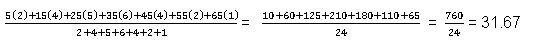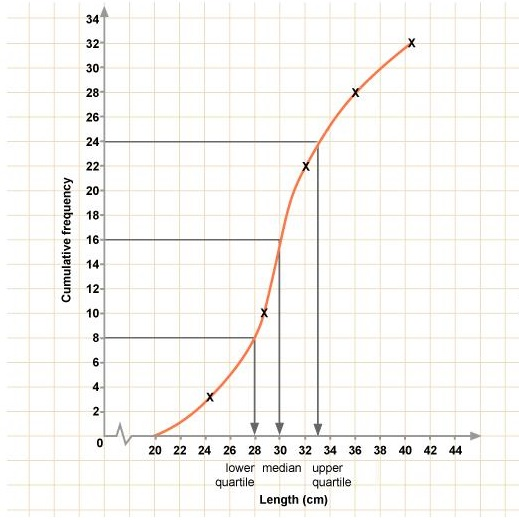# GCSE Maths Unit 1 - Frequency Distribution Tables

##### March 3, 2016

GCSE Maths: Frequency Distribution Tables and Cumulative Frequency

The modal class is the class interval with the highest frequency. To get the mean of a distribution, we multiply each value by its frequency; add these together and divide by the total of the frequencies.

Example

Find the mode and mean of the distribution summarised in the table below.

 Value 5 15 25 35 45 55 65 Frequency 2 4 5 6 4 2 1

Mode = 35 (it has the highest frequency 6)

Mean =A cumulative frequency distribution table is similar to a normal frequency table except that we continuously add our frequencies to give a frequency for that class or lower. Once the cumulative frequencies have been plotted, both the median and the quartiles (half-way between the median and the ends, i.e.  1/4 or 3/4 - way through the data) can be easily read from the graph.Example

i) Convert the frequency distribution table below into a cumulative frequency distribution table and then plot the data as a cumulative frequency graph.

 Length 24 28 32 36 40 Frequency 3 7 12 6 4

ii) Use this graph to determine the median and both the lower and the upper quartiles of this data.

 Length 24 28 32 36 40 Frequency 3 7 12 6 4 Cumulative Frequency 3 10 (7+3) 22 (10+12) 28 (22+6) 32 (28+4)Solutions

Total cumulative frequency = 32

Lower Quartile @ frequency of 1/4 of the total

1/4 x 32 = 8, which corresponds to a length of 28

Median @ frequency of 1/2 of the total

1/2 x 32 = 16, which corresponds to a length of 30

Upper Quartile @ frequency of 3/4 of the total

3/4 x 32 = 24, which corresponds to a length of 33

### Work Towards That 'A' Grade

Achieve the results you want with-written revision resources when you register with iRevise.
Its Free!